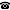# 2019 seminar talk: Isometries of combinatorial Banach spaces

Talk held by Christina Brech (Universidade de São Paulo, Brazil) at the KGRC seminar on 2019-10-10.

### Abstract

The Schreier family $\mathcal{S} = \{F \in [\omega]^{<\omega}: |F| \leq \min+1\}$ induces a structure with no infinite sets of indiscernibles and this can be generalized to the context of Banach spaces. The fact that the canonical basis of the Banach space induced by the Schreier family doesn't have infinite indiscernibles gives a hint on the rigidity of this object, that is, on the fact that isometries of the space fix the basis, up to signs.

In this talk, we will give the background for the previous paragraph and will present a generalized version of it, obtained in a joint work with V. Ferenczi and A. Tcaciuc: given a regular family $\mathcal{F}$, it is possible to define its corresponding combinatorial space $X_\mathcal{F}$, which is a Banach space whose norm is defined in terms of the family $\mathcal{F}$. We prove that every isometry of a combinatorial Banach space $X_\mathcal{F}$ is induced by a signed permutation of its canonical basis.

Kurt Gödel Research Center for Mathematical Logic. Währinger Straße 25, 1090 Wien, Austria.+43-1-4277-50501. Last updated: 2010-12-16, 04:37.